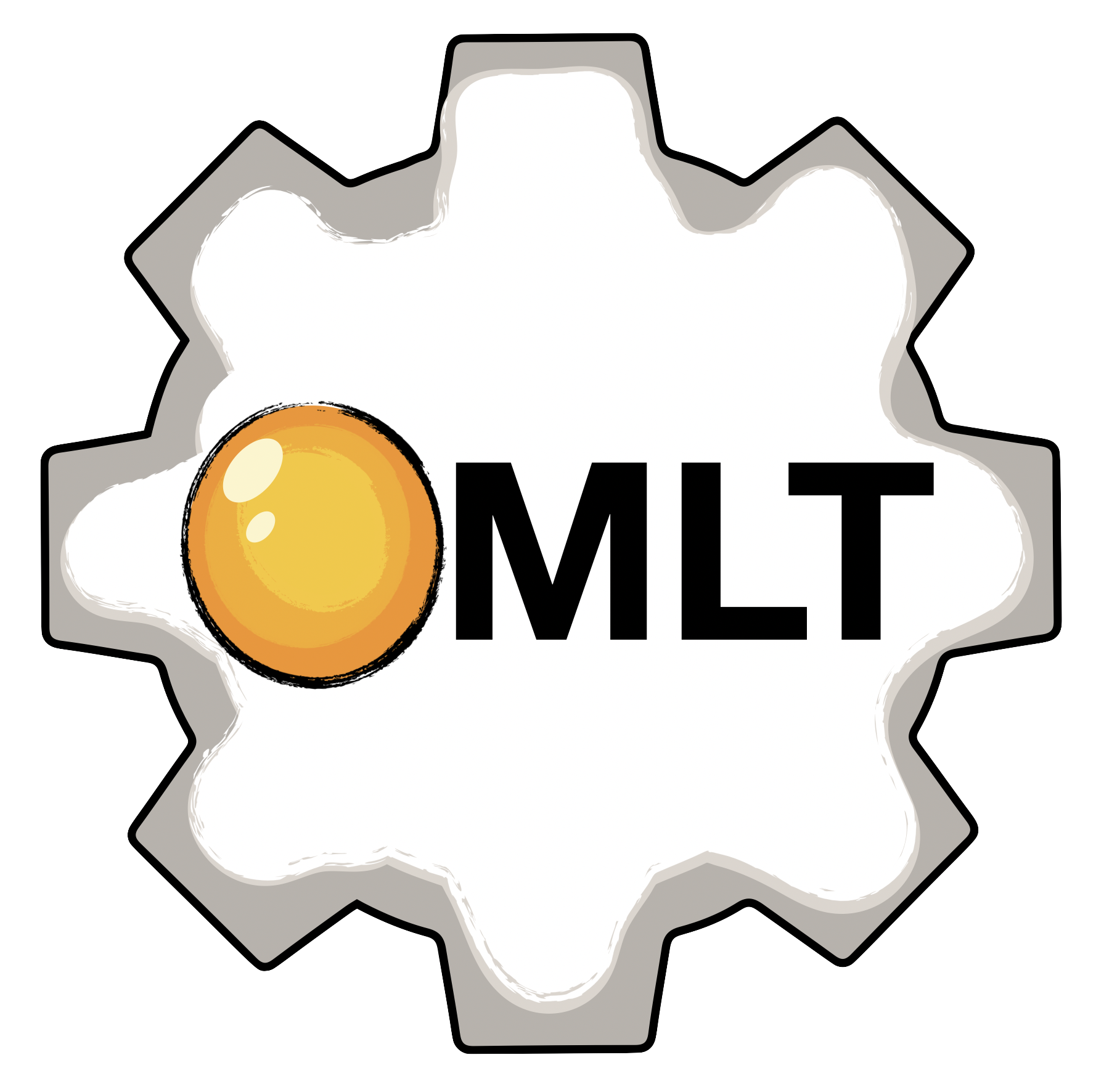# OMLT: Optimization and Machine Learning Toolkit#

OMLT is a Python package for representing machine learning models (neural networks and gradient-boosted trees) within the Pyomo optimization environment. The package provides various optimization formulations for machine learning models (such as full-space, reduced-space, and MILP) as well as an interface to import sequential Keras and general ONNX models.

## Examples#

```import tensorflow
import pyomo.environ as pyo
from omlt import OmltBlock, OffsetScaling
from omlt.neuralnet import FullSpaceNNFormulation, NetworkDefinition

#create a Pyomo model with an OMLT block
model = pyo.ConcreteModel()
model.nn = OmltBlock()

#the neural net contains one input and one output
model.input = pyo.Var()
model.output = pyo.Var()

#apply simple offset scaling for the input and output
scale_x = (1, 0.5)       #(mean,stdev) of the input
scale_y = (-0.25, 0.125) #(mean,stdev) of the output
scaler = OffsetScaling(offset_inputs=[scale_x],
factor_inputs=[scale_x],
offset_outputs=[scale_y],
factor_outputs=[scale_y])

#provide bounds on the input variable (e.g. from training)
scaled_input_bounds = {0:(0,5)}

#load the keras model into a network definition

#multiple formulations of a neural network are possible
#this uses the default NeuralNetworkFormulation object
formulation = FullSpaceNNFormulation(net)

#build the formulation on the OMLT block
model.nn.build_formulation(formulation)

#query inputs and outputs, as well as scaled inputs and outputs
model.nn.inputs
model.nn.outputs
model.nn.scaled_inputs
model.nn.scaled_outputs

#connect pyomo model input and output to the neural network
@model.Constraint()
def connect_input(mdl):
return mdl.input == mdl.nn.inputs

@model.Constraint()
def connect_output(mdl):
return mdl.output == mdl.nn.outputs

#solve an inverse problem to find that input that most closely matches the output value of 0.5
model.obj = pyo.Objective(expr=(model.output - 0.5)**2)
status = pyo.SolverFactory('ipopt').solve(model, tee=False)
print(pyo.value(model.input))
print(pyo.value(model.output))
```

## Development#

OMLT uses tox to manage development tasks:

• tox -av to list available tasks

• tox to run tests

• tox -e lint to check formatting and code styles

• tox -e format to automatically format files

• tox -e docs to build the documentation

• tox -e publish to publish the package to PyPi

## Contributors#

GitHub

Name

AcknowledgementsJordan Jalving

This work was funded by Sandia National Laboratories, Laboratory Directed Research and Development programFrancesco Ceccon

This work was funded by an Engineering & Physical Sciences Research Council Research Fellowship [GrantNumber EP/P016871/1]Carl D. Laird

Initial work was funded by Sandia National Laboratories, Laboratory Directed Research and Development program. Current work supported by Carnegie Mellon University.Calvin Tsay

This work was funded by an Engineering & Physical Sciences Research Council Research Fellowship [GrantNumber EP/T001577/1], with additional support from an Imperial College Research Fellowship.Alexander Thebelt

This work was supported by BASF SE, Ludwigshafen am Rhein.